# Getting Started

This vignette offers a few basic examples to help users get started with plotmm.

The package has five functions:

1. plot_mm(): The main function of the package, plot_mm allows the user to simply input the name of the fit mixture model, as well as an optional argument to pass the number of components k that were used in the original fit. Note: the function will automatically detect the number of components if k is not supplied. The result is a tidy ggplot of the density of the data with overlaid mixture weight component curves. Importantly, as the grammar of graphics is the basis of visualization in this package, all other tidyverse-friendly customization options work with any of the plotmm’s functions (e.g., customizing with ggplot2’s functions like labs() or theme_*(); or patchwork’s plot_annotation()). There are examples of these and others below.

2. plot_cut_point(): Mixture models are often used to derive cut points of separation between groups in feature space. plot_cut_point() plots the data density with the overlaid cut point (the mean of the calculated mu) from the fit mixture model.

3. plot_mix_comps(): A helper function allowing for expanded customization of mixture model plots. The function superimposes the shape of the components over a ggplot2 object. This function is also used to render all plots in the main plot_mm() function.

4. plot_gmm(): The original function upon which the package was expanded. It is included in plotmm for quicker access to a common mixture model form (univariate Gaussian), as well as to bridge between the original plotGMM package.

5. plot_mix_comps_normal(): Similarly, this function is the original basis of plot_mix_comps(), but for Gaussian mixture models only. It is included in plotmm for bridging between the original plotGMM package.

The package supports several model objects (from ‘mixtools’, ‘EMCluster’, and ‘flexmix’), as well as many mixture model specifications, including mixtures of:

1. Univariate Gaussians
2. Bivariate Gaussians
3. Gammas
4. Logistic regressions
5. Linear regressions
6. Poisson regressions

First, load the stable version from CRAN.

library(plotmm)

### Tidy visualization of mixture models via plot_mm()

First, here is an example for univariate normal mixture model:

library(mixtools)
#> mixtools package, version 1.2.0, Released 2020-02-05
#> This package is based upon work supported by the National Science Foundation under Grant No. SES-0518772.
library(ggplot2)

set.seed(576)

mixmdl <- normalmixEM(iris$Petal.Length, k = 2) #> number of iterations= 9 # visualize plot_mm(mixmdl, 2) + labs(title = "Univariate Gaussian Mixture Model", subtitle = "Mixtools Object")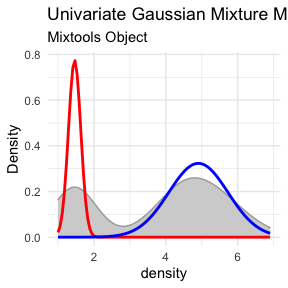Next is an example of a mixture of linear regressions: library(mixtools) library(ggplot2) # set up the data (replication of mixtools examples for comparability) data(NOdata) attach(NOdata) set.seed(100) out <- regmixEM(Equivalence, NO, verb = TRUE, epsilon = 1e-04) #> iteration= 1 diff= 61.52481 log-likelihood 59.2794 #> iteration= 2 diff= 15.93577 log-likelihood 75.21517 #> iteration= 3 diff= 27.66707 log-likelihood 102.8822 #> iteration= 4 diff= 17.24268 log-likelihood 120.1249 #> iteration= 5 diff= 1.238034 log-likelihood 121.363 #> iteration= 6 diff= 0.2725981 log-likelihood 121.6356 #> iteration= 7 diff= 0.1762554 log-likelihood 121.8118 #> iteration= 8 diff= 0.1116986 log-likelihood 121.9235 #> iteration= 9 diff= 0.06262589 log-likelihood 121.9861 #> iteration= 10 diff= 0.03058315 log-likelihood 122.0167 #> iteration= 11 diff= 0.0132683 log-likelihood 122.03 #> iteration= 12 diff= 0.005278647 log-likelihood 122.0353 #> iteration= 13 diff= 0.001982899 log-likelihood 122.0372 #> iteration= 14 diff= 0.0007186432 log-likelihood 122.038 #> iteration= 15 diff= 0.0002548647 log-likelihood 122.0382 #> iteration= 16 diff= 8.922635e-05 log-likelihood 122.0383 #> number of iterations= 16 df <- data.frame(out$beta)

# visualize
plot_mm(out) +
labs(title = "Mixture of Regressions",
subtitle = "Mixtools Object")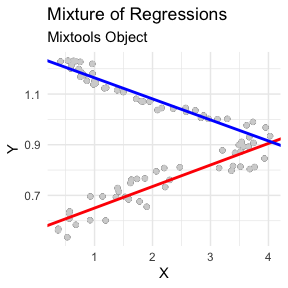Next is a bivariate Gaussian mixture model (via EMCluster). Note: in this case, all plots print by default for full display of options. Use indexing (e.g., plot) to plot a specific or preferred quantity.

library(EMCluster)
library(patchwork)
#>
#> Attaching package: 'patchwork'
#> The following object is masked from 'package:MASS':
#>
#>     area

set.seed(1234)

x <- da1$da out <- init.EM(x, nclass = 10, method = "em.EM") plot_mm(out, data = x) + plot_annotation(title = "Bivariate Gaussian Mixture Model", subtitle = "EMCluster Object")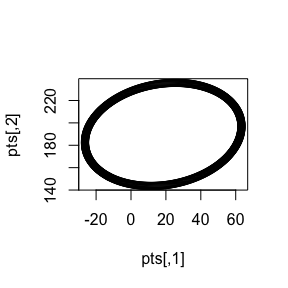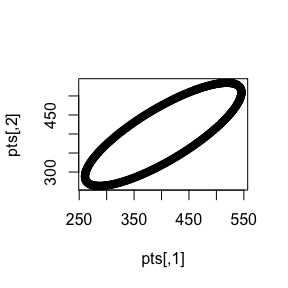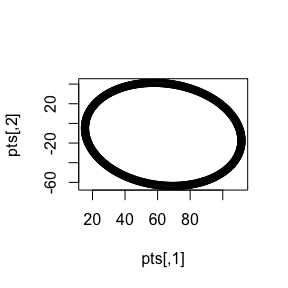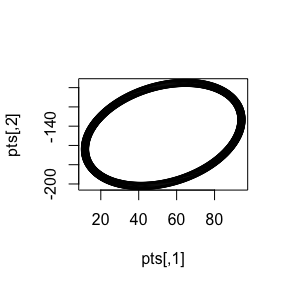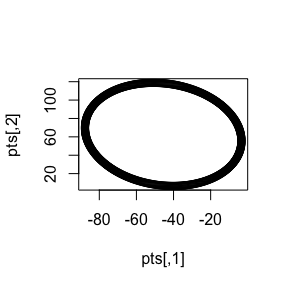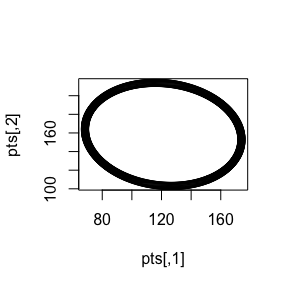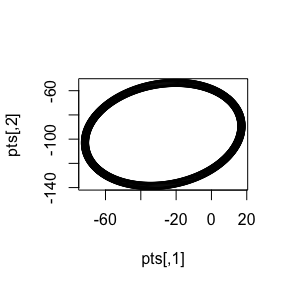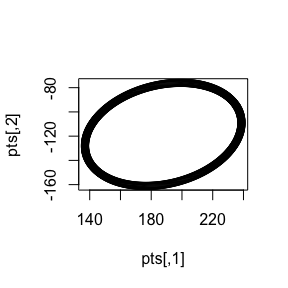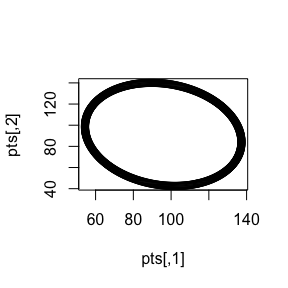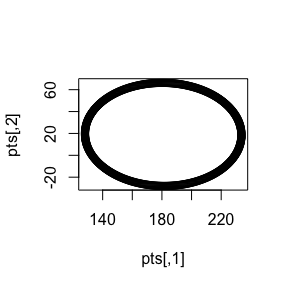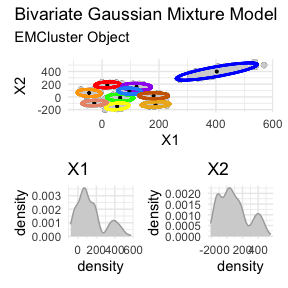### Plot cut points (or not) via plot_cut_point() (with the amerika color palette) library(mixtools) mixmdl <- normalmixEM(faithful$waiting, k = 2)
#> number of iterations= 52

plot_cut_point(mixmdl, plot = TRUE, color = "amerika") # produces plot
#> Registered S3 method overwritten by 'wesanderson':
#>   method        from
#>   print.palette amerika
#> stat_bin() using bins = 30. Pick better value with binwidth.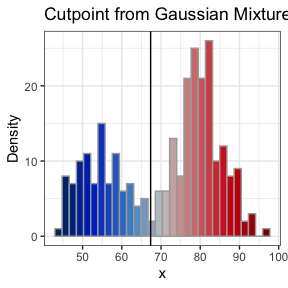plot_cut_point(mixmdl, plot = FALSE) # gives the cut point value, not the plot
#>  67.35299

### Customize a ggplot with plot_mix_comps()

library(mixtools)
library(magrittr)
library(ggplot2)

# Fit a univariate mixture model via mixtools
set.seed(576)

mixmdl <- normalmixEM(faithful$waiting, k = 2) #> number of iterations= 24 # Customize a plot with plot_mix_comps_normal() data.frame(x = mixmdl$x) %>%
ggplot() +
geom_histogram(aes(x, ..density..), binwidth = 1, colour = "black",
fill = "white") +
stat_function(geom = "line", fun = plot_mix_comps_normal, # here is the function
args = list(mixmdl$mu, mixmdl$sigma, lam = mixmdl$lambda), colour = "red", lwd = 1.5) + stat_function(geom = "line", fun = plot_mix_comps_normal, # here again as k = 2 args = list(mixmdl$mu, mixmdl$sigma, lam = mixmdl$lambda),
colour = "blue", lwd = 1.5) +
ylab("Density")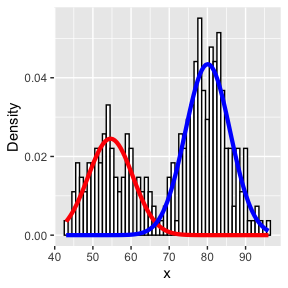## Contribute

Anyone is welcome to contribute to the package. Before collaborating, please take a look at and abide by the contributor code of conduct. Here’s a sampling of how to contribute:

• Submit an issue reporting a bug, requesting a feature enhancement, etc.

• Suggest changes directly via pull request

• Reach out directly with ideas if you’re uneasy with public interaction